Download Presentation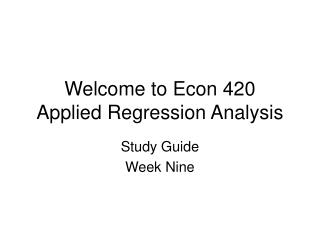Welcome to Econ 420 Applied Regression Analysis

# Welcome to Econ 420 Applied Regression Analysis - PowerPoint PPT Presentation

Welcome to Econ 420 Applied Regression Analysis. Study Guide Week Nine. Some of you did not do as well as I expected on Assignment 2. So here is what we will do. I explain the assignment. You will redo Question 3 & 4 plus a couple of other questions.I am the owner, or an agent authorized to act on behalf of the owner, of the copyrighted work described.
Download Presentation## Welcome to Econ 420 Applied Regression Analysis

An Image/Link below is provided (as is) to download presentation

Download Policy: Content on the Website is provided to you AS IS for your information and personal use and may not be sold / licensed / shared on other websites without getting consent from its author.While downloading, if for some reason you are not able to download a presentation, the publisher may have deleted the file from their server.

- - - - - - - - - - - - - - - - - - - - - - - - - - E N D - - - - - - - - - - - - - - - - - - - - - - - - - -
Presentation Transcript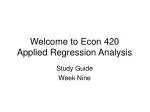### Welcome to Econ 420 Applied Regression Analysis

Study Guide

Week Nine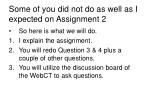Some of you did not do as well as I expected on Assignment 2
• So here is what we will do.
• I explain the assignment.
• You will redo Question 3 & 4 plus a couple of other questions.
• You will utilize the discussion board of the WebCT to ask questions.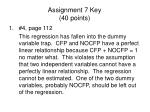Assignment 7 Key (40 points)
• #4, page 112

This regression has fallen into the dummy variable trap. CFP and NOCFP have a perfect linear relationship because CFP + NOCFP = 1 no matter what. This violates the assumption that two independent variables cannot have a perfectly linear relationship. The regression cannot be estimated. One of the two dummy variables, probably NOCFP, should be left out of the regression.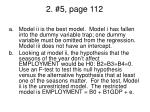2. #5, page 112

a. Model ii is the best model. Model i has fallen into the dummy variable trap; one dummy variable must be omitted from the regression. Model iii does not have an intercept.

b. Looking at model ii, the hypothesis that the seasons of the year don’t affect EMPLOYMENT would be H0: B2=B3=B4=0. Use an F-test to test this null hypothesis versus the alternative hypothesis that at least one of the seasons matter. For the test, Model ii is the unrestricted model. The restricted model is EMPLOYMENT = B0 + B1GDP + e.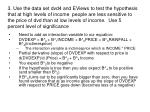3. Use the data set dvd4 and EViews to test the hypothesis that at high levels of income people are less sensitive to the price of dvd than at low levels of income. Use 5 percent level of significance

• Need to add an interaction variable to our equation:
• DVDEXP = B^0 + B^1INCOME + B^2PRICE + B^3RAINFALL + B^4(inctimesprice)
• The interaction variable is inctimesprice which is INCOME * PRICE
• Partial derivative (slope) of DVDEXP with respect to price is
• d(DVDEXP)/d (Price) = B^2 + B^4 Income
• You expect B^2to be negative
• If the hypothesis is true then you also expect B^4 to be positive (and smaller than B^2)
• If B^4 turns out to be significantly bigger than zero, then you have found evidence that at as income goes up the slope of DVDEXP with respect to PRICE goes down (becomes less of a negative)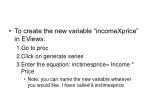To create the new variable “incomeXprice” in EViews:
• Go to proc
• Click on generate series
• Enter the equation: inctimesprice= Income * Price
• Note: you can name the new variable whatever you would like. I have called it inctimesprice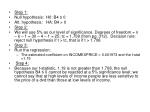Step 1:
• Null hypothesis: H0: B4 ≤ 0
• Alt. hypothesis: HA: B4 > 0
• Step 2:
• We will use 5% as our level of significance. Degrees of freedom = n – k – 1 = 30 – 4 – 1 = 25; tc = 1.708 (from pg. 312). Decision rule: reject null hypothesis if t > tc, that is if t > 1.708
• Step 3:
• Run the regression:
• The estimated coefficient on INCOMEXPRICE = 0.001973 and the t-stat =1.19
• Step 4:
• Because our t-statistic, 1.19 is not greater than 1.708, the null hypothesis B4 ≤ 0 cannot be rejected at a 5% significance level; we cannot say that at high levels of income people are less sensitive to the price of a dvd than those at low levels of income.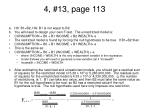4, #13, page 113

a. H0: B1=B2, HA: B1 is not equal to B2

b. You will need to design your own F-test. The unrestricted model is:

• CONSUMPTION = B0 + B1 INCOME + B2 WEALTH+ e
• The restricted model is found by forcing the null hypothesis to be true. If B1=B2 then
• CONSUMPTION = B0 + B1 INCOME + B1 WEALTH+ e
• This is the same as:
• CONSUMPTION = B0 + B1 (INCOME + WEALTH) + e
• where (INCOME + WEALTH) is the only independent variable in the regression.
• Under EViews you will need to generate a new variable call it “incwealth”
• Incwealth = INCOME + WEALTH

c. After estimating the restricted and unrestricted models, you should get a residual sum of squares for the restricted model of 5.28 x 109 or 5,280,000,000. The residual sum of squares for the unrestricted model is 4.33 x 109 or 4,330,000,000. q, the number of restrictions, is 1. B1 can take any value, and then B2 must take the same value as B1 for the null hypothesis to be true, so B2 has a restricted value if the null hypothesis is true. The null hypothesis used here only imposes one restriction.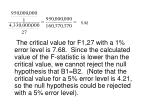5.92

The critical value for F1,27 with a 1% error level is 7.68. Since the calculated value of the F-statistic is lower than the critical value, we cannot reject the null hypothesis that B1=B2. (Note that the critical value for a 5% error level is 4.21, so the null hypothesis could be rejected with a 5% error level).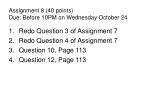• Redo Question 3 of Assignment 7
• Redo Question 4 of Assignment 7
• Question 10, Page 113
• Question 12, Page 113